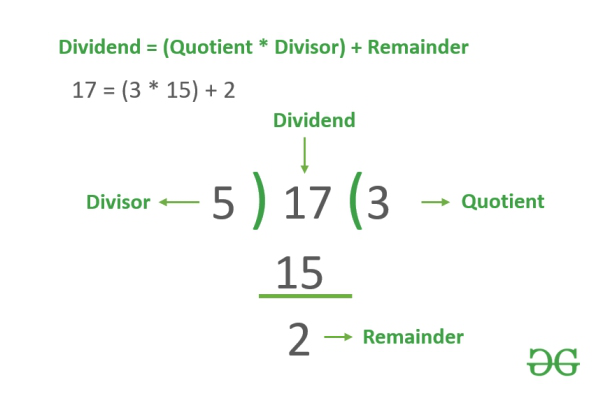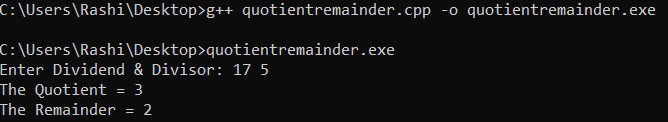# C++ Program to Find Quotient and Remainder

• Last Updated : 19 Jul, 2022

Here, we will see how to find the quotient and remainder using a C++ program.Examples:

Input: Dividend = 17, Divisor = 5
Output: Quotient = 3, Remainder = 2

Input: Dividend = 23, Divisor = 3
Output: Quotient = 7, Remainder = 2

The Modulo Operator will be used to calculate the Remainder and to calculate the Quotient the Division Operator will be used.

Quotient = Dividend / Divisor;

Remainder = Dividend % Divisor;

Below is the C++ program to find the quotient and remainder:

## C++

 `// C++ program to find quotient``// and remainder``#include``using` `namespace` `std;` `// Driver code``int` `main()``{``    ``int` `Dividend, Quotient,``        ``Divisor, Remainder;` `    ``cout << ``"Enter Dividend & Divisor: "``;``    ``cin >> Dividend >> Divisor;` `    ``Quotient = Dividend / Divisor;``    ``Remainder = Dividend % Divisor;` `    ``cout << ``"The Quotient = "` `<<``             ``Quotient << endl;``    ``cout << ``"The Remainder = "` `<<``             ``Remainder << endl;``    ``return` `0;``}`

Output :Time Complexity: O(1)
Auxiliary Space: O(1)

Note: If Divisor is ‘0’ then it will be showing an ArithmeticException Error.

My Personal Notes arrow_drop_up